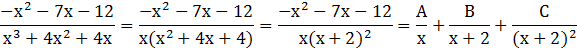### Sample Problem

Write the partial fraction decomposition of.

#### Solution

Long division gives youMultiply each side by x(x+2)2 [the least common denominator]

-x2-7x-12=A(x+2)2+Bx(x+2)+Cx

This equation is true for all x so we can substitute any number for x.

Let’s choose x=-2 and x=0 for convenience.

When x=-2

-(-2)2-7(-2)-12=C(-2)

-4+14-12=-2C

1=C

When x=0

-(0)2-7(0)-12=A(0+2)2+B(0)(0+2)+C(0)

-12=4A

A=-3

Use x=1, A=-3, C=1 to find the value of B

-x2-7x-12=A(x+2)2+Bx(x+2)+Cx

-(1)2-7(1)-12=-3(1+2)2+B(1)(1+2)+(1)(1)

-20=-27+3b+1

-20=-26+3b

6=3b

B=2

So, the partial fraction decomposition is (don’t forget the remainder from your long division!):